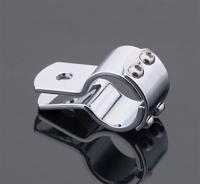# Find Your Clamp SizeThis question is asked a lot on motorcycle forums and lists. "What size clamp do I need to attach my (light, highway peg, speedometer cable, clutch cable etc) to my (frame, dresser bar etc)".

We're all supposed to remember our grammar school math that tells us to use the formula circumference / pi = diameter. Oh sure, we all remember that stuff, use it every day. Yeah right. Well here's a simple calculator that'll get the job done for you.

Go to your bike with a piece of string or strip of paper and wrap the string/paper around whatever round object you want to put a clamp on. If you end up with an odd number like 4 1/2" convert to decimel 4.5 and enter it in the "Circumference" box below then click on the "Solve" box. You'll be shown the diameter of the clamp you need. Don't remember how to convert a fraction to a decimal? Just divide the numerator (top number) by the denominator (bottom number) on the handy dandy calculator provided below so 3/16" would be .18, 7/8" would be .87 etc.

## Let's Do This

Take your measurement with tape or string and if there's a fraction enter the numerator in the first box, denominator in the second box then click on the 'divide' ( / ) button to get the decimal equivalent if there is one.

Example: the Nomad engine protector bar is 3 15/16". Enter the 15 and the 16 in the boxes below and click on /.

=

Now enter your whole number plus the decimal (if any) in the "Circumference" box and click the "Solve" button:

 Area: Diameter: Circumference:

The number you see in the "Diameter" box is the clamp size you need for your project.

Go Ride,
Gadget

## Share It

Be the first to comment